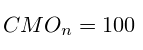# The indicator of price points Chande

The following describes the calculator indicator of price points Chande, and builds a graph by candles.

Chande Momentum Oscillator (CMO) - this is the indicator. And it is called so because evo invented scientist Tushar Chande.

The function of the indicator is to count the movement of the price for a certain number of periods.

For example, for 2 adjacent spark calculator takes the difference, suppose the value of the penultimate and last closing candles. If the difference is positive - then the price went up, the further it is added on to the total positive difference, and if on the contrary, a negative difference - the cost of the value will go down, and then added on to the total negative difference.

The formula for calculating the indicator is as follows:Of course, the schedule will jump from -100 to +100. And the essence of the indicator is as follows: if the value rises above 50 or below -50, it means that something is bought or on the contrary a lot, a lot of selling.

﻿

Indicator price moments Changde
Moving secondary:
Moving secondary:
Candles for USDJPY
Import data
For separation fields can use one from these characters: Tab, ";" or ",":